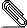# Re: [HACKERS] parallelize queries containing initplans

```On Sun, Aug 13, 2017 at 6:49 PM, Haribabu Kommi
<kommi.harib...@gmail.com> wrote:
> On Fri, Aug 11, 2017 at 1:18 AM, Amit Kapila <amit.kapil...@gmail.com>
> wrote:
>>
>
> Thanks for the updated patch. Patch looks fine. I just have some
>
> + * ExecEvalParamExecParams
> + *
> + * Execute the subplan stored in PARAM_EXEC initplans params, if not
> executed
> + * till now.
> + */
> +void
> +ExecEvalParamExecParams(Bitmapset *params, EState *estate)
>
> I feel it is better to explain when this function executes the sub plans
> that are
> not executed till now? Means like in what scenario?
>```
```
It just means that it will execute the same initplan (subplan) just
once in master backend even if it used in multiple places.  This is
the same kind of usage as we have in ExecEvalParamExec.  You can find
its usage by using some query like

explain analyse select sum(t1.i) from t1, t2 where t1.j=t2.j and t1.k
= (select count(k) from t3) and t1.k=t2.k;

Ensure you insert some rows in t1 and t2 that match the count from t3.
If you are using the schema and data given in Kuntal's script in email
above, then you need to insert something like
t1(900,900,900);t2(900,900,900);

It generates plan like below:

postgres=# explain analyse select sum(t1.i) from t1, t2 where
t1.j=t2.j and t1.k = (select count(k) from t3) and t1.k=t2.k;
QUERY PLAN
-----------------------------------------------------------------------------------------------------------------------------------
Aggregate  (cost=29.65..29.66 rows=1 width=8) (actual
time=22572.521..22572.521 rows=1 loops=1)
InitPlan 1 (returns \$0)
->  Finalize Aggregate  (cost=9.70..9.71 rows=1 width=8) (actual
time=4345.110..4345.111 rows=1 loops=1)
->  Gather  (cost=9.69..9.70 rows=2 width=8) (actual
time=4285.019..4345.098 rows=3 loops=1)
Workers Planned: 2
Workers Launched: 2
->  Partial Aggregate  (cost=9.69..9.70 rows=1
width=8) (actual time=0.154..0.155 rows=1 loops=3)
->  Parallel Seq Scan on t3  (cost=0.00..8.75
rows=375 width=4) (actual time=0.011..0.090 rows=300 loops=3)
->  Nested Loop  (cost=0.00..19.93 rows=1 width=4) (actual
time=22499.918..22572.512 rows=1 loops=1)
Join Filter: (t1.j = t2.j)
->  Gather  (cost=0.00..9.67 rows=2 width=12) (actual
time=10521.356..10521.363 rows=1 loops=1)
Workers Planned: 2
Params Evaluated: \$0
Workers Launched: 2
->  Parallel Seq Scan on t1  (cost=0.00..9.67 rows=1
width=12) (actual time=0.506..0.507 rows=0 loops=3)
Filter: (k = \$0)
Rows Removed by Filter: 299
->  Materialize  (cost=0.00..10.21 rows=2 width=8) (actual
time=11978.557..12051.142 rows=1 loops=1)
->  Gather  (cost=0.00..10.20 rows=2 width=8) (actual
time=11978.530..12051.113 rows=1 loops=1)
Workers Planned: 2
Params Evaluated: \$0
Workers Launched: 2
->  Parallel Seq Scan on t2  (cost=0.00..10.20
rows=1 width=8) (actual time=0.067..0.067 rows=0 loops=3)
Filter: (k = \$0)
Rows Removed by Filter: 333
Planning time: 15103.237 ms
Execution time: 22574.703 ms
(27 rows)

You can notice that initplan is used at multiple nodes, but it will be
evaluated just once.  If you want, I can add a sentence in the
comments, but I think this is somewhat obvious and the same use case
already exists.  Let me know if you still think that comments need to
be expanded?

>
> + if (params == NULL)
> + nparams = 0;
> + else
> + nparams = bms_num_members(params);
>
> bms_num_members return 0 in case if the params is NULL.
> Is it fine to keep the specific check for NULL is required for performance
> benefit
> or just remove it? Anything is fine for me.
>

I don't see any performance benefit here, so removed the if check.

>
> + if (IsA(plan, Gather))
> + ((Gather *) plan)->initParam = bms_intersect(plan->lefttree->extParam,
> initSetParam);
> + else
> + ((GatherMerge *) plan)->initParam =
> bms_intersect(plan->lefttree->extParam, initSetParam);
>
>
> I think the above code is to find out the common parameters that are prsent
> in the external
> and out params.
>

Here, we want to save all the initplan params that can be used below
the gather node.  extParam contains the set of all external PARAM_EXEC
params that can be used below gather node and we just want initplan
params out of those.

> It may be better to explain the logic in the comments.
>

I have kept comments atop of the function set_param_references to
explain the context, but now I have added few more in the code as
suggested by you.  See if that suffices the need.

--
With Regards,
Amit Kapila.
EnterpriseDB: http://www.enterprisedb.com
```pq_pushdown_initplan_v7.patch
Description: Binary data

```--
Sent via pgsql-hackers mailing list (pgsql-hackers@postgresql.org)
To make changes to your subscription:
http://www.postgresql.org/mailpref/pgsql-hackers
```